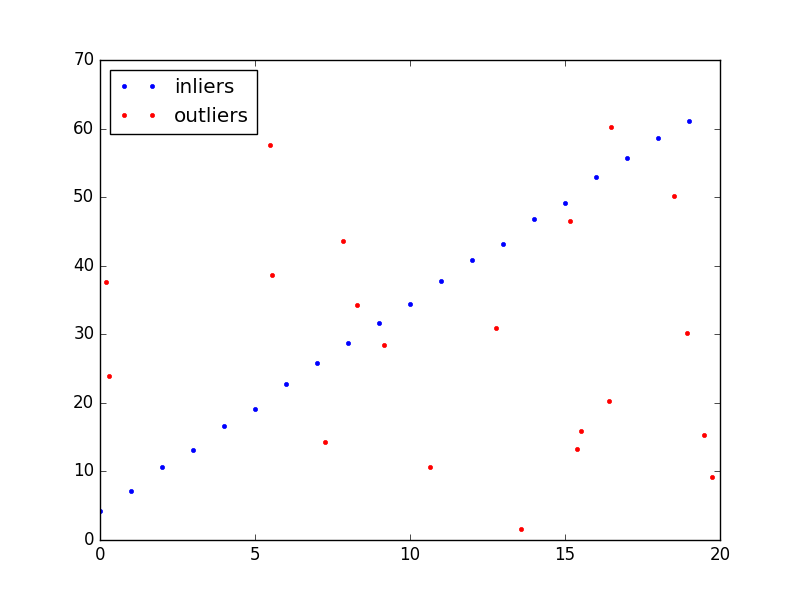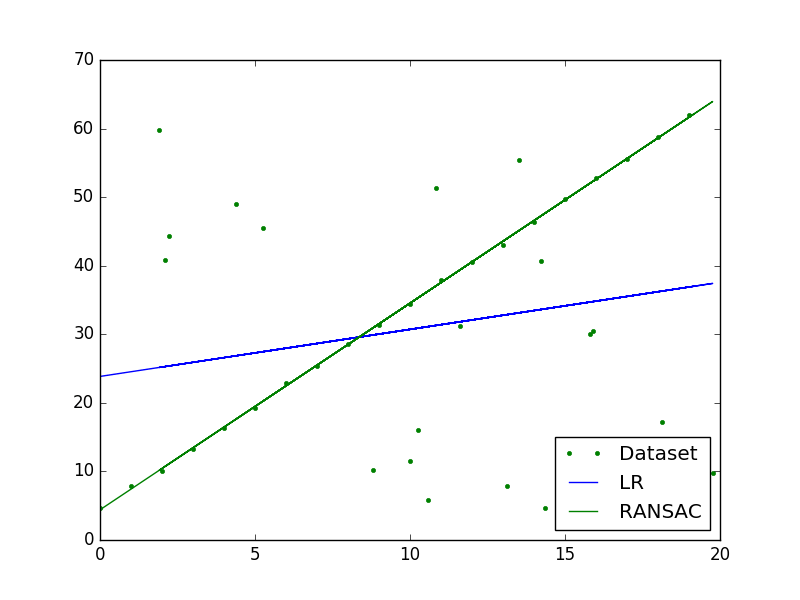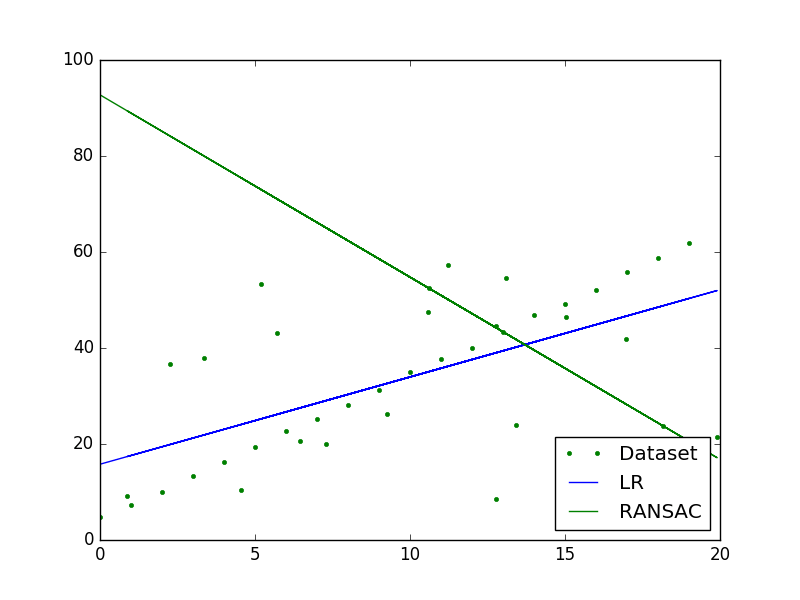# NIUHE

## Algorithm

1. 从全部数据集中随机抽取一小部分，我们假设这部分数据都是正常的，用这部分数据训练模型。采样集的大小是能训练模型的最小样本数。
2. 用这个模型测试其他剩余样本，如果某个样本的损失小于某个阈值，则认为它是和抽样出的样本是一致的，即也是正常样本；若某个样本的损失大于阈值，则认为它是异常样本。

1. 从原始数据中采样出一个子集，假设为正常样本集。
2. 用上述子集训练模型
3. 用其他样本测试该模型。对于拟合很好的样本点，可以通过损失小于某一阈值来判断，把它加入一致集。
4. 如果一致集的样本有足够多的样本，则认为该模型很好，拟合了大部分正常样本。
5. 然后，用一致集和采样集中的样本重新训练模型，这也许会进一步提升模型的效果，如果整体损失比上次迭代小，则更新最佳拟合模型。
6. 重复步骤1～5，直到一致集样本足够多或者达到最大迭代次数，返回最佳拟合模型。

Python代码：

## DEMO## Parameters

RANSAC算法中有几个超参数需要调试：

• t, d : 这两个参数需要根据应用和数据集来调节

• k（最大迭代次数）：可以从理论上确定。

定义 $p$ 是RANSAC算法在某次迭代采样时只采样到正常样本的概率。如果这真的发生了，那么这次迭代将训练出一个很好的模型，所以 $p$ 也代表了RANSAC算法得出一个好结果的概率。再定义 $w$ 为每次采样时，采到一个正常点的概率，即 $w$ =  number of inliers in data / number of points in data。假设一次采样选取 $n$ 的样本，那么这次采样选择的样本全部都是正常样本的概率为 $w^n$（假设为放回采样），至少选择一个异常样本的概率就为 $1-w^n$。总共迭代 $k$ 次，那么这 $k$ 次采样都至少有一个异常样本的概率为：$(1-w^n)^k$，应该等于 $1-p$，即 $1-p=(1-w^n)^k$ 两边同时取对数得： $k = \frac{\log(1-p)}{\log(1-w^n)}$ 实际上我们是不放回采样，所以还要在上面的基础上加上 $k$ 的标准差。$k$ 的标准差定义为： $SD(k) = \frac{\sqrt{1-w^n}}{w^n}$

• 没有时间上界，即不会自然收敛，只能靠最大迭代次数限制。有时可能最后一次迭代才找到一个好的模型，有时可能第一次就找到了

• 需要根据不同问题手动调试参数（一些阈值）

• 只能同时评估一种模型

• 不是所有时候都能找到合适的模型，尤其是在异常样本个数大于 50% 的时候，如下图所示：## 参考

Random sample consensus